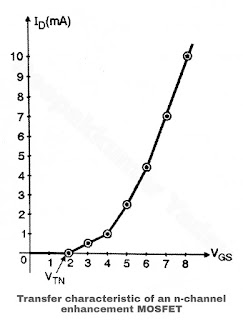# Transfer Characteristics of n-channel Enhancement Type MOSFET

Transfer Characteristics of n-channel Enhancement Type MOSFET

• The transfer characteristics of a n-channel enhancement MOSFET are as shown in Figure.
• Note that the drain current is zero for VGS ≤ VTN For an n-channel E-MOSFET the threshold voltage is denoted by VTN and for a p-channel E-MOSFET the threshold voltage is denoted by VTP.
• The transfer characteristics shown in Figure is a characteristic of VGS Vs ID. It is now totally in the positive VGS region and remains zero till VGS = VTN.
• The relation between drain current and VGS is given by the following equation,
I= k (VGS - VTN)2

• Here k is a constant and its value depends on the construction of the device.
• For n-channel E-MOSFETS the constant is sometimes denoted by kn and for a p-channel E-MOSFET, it is denoted by kp.
• The expression for ID is a nonlinear relation and it is valid only for VGS > VTN. Therefore the transfer characteristics is also nonlinear.